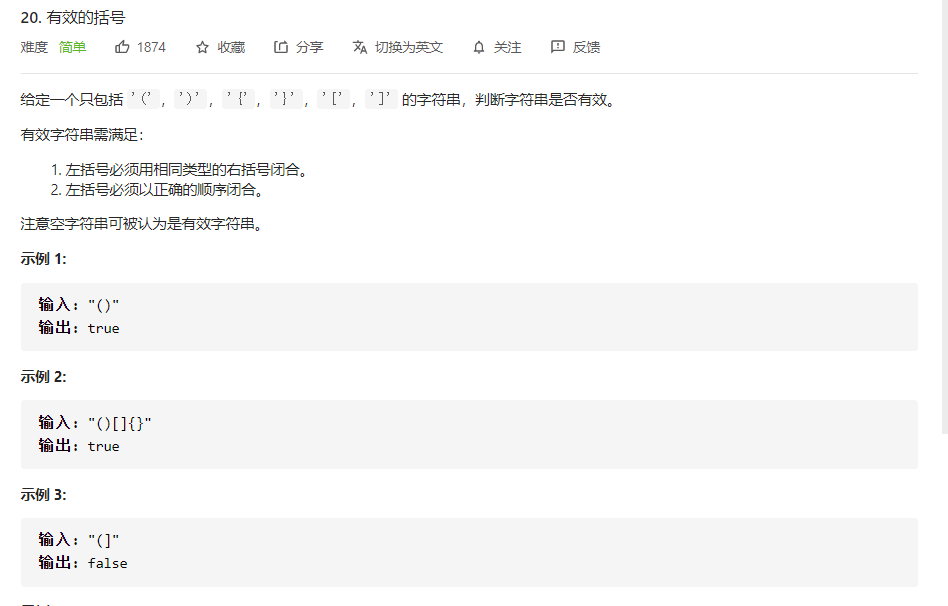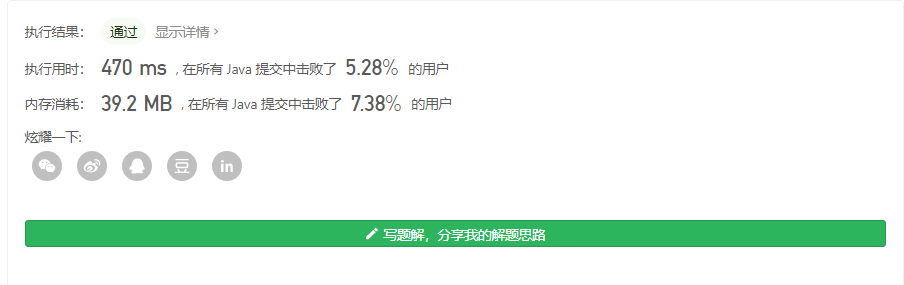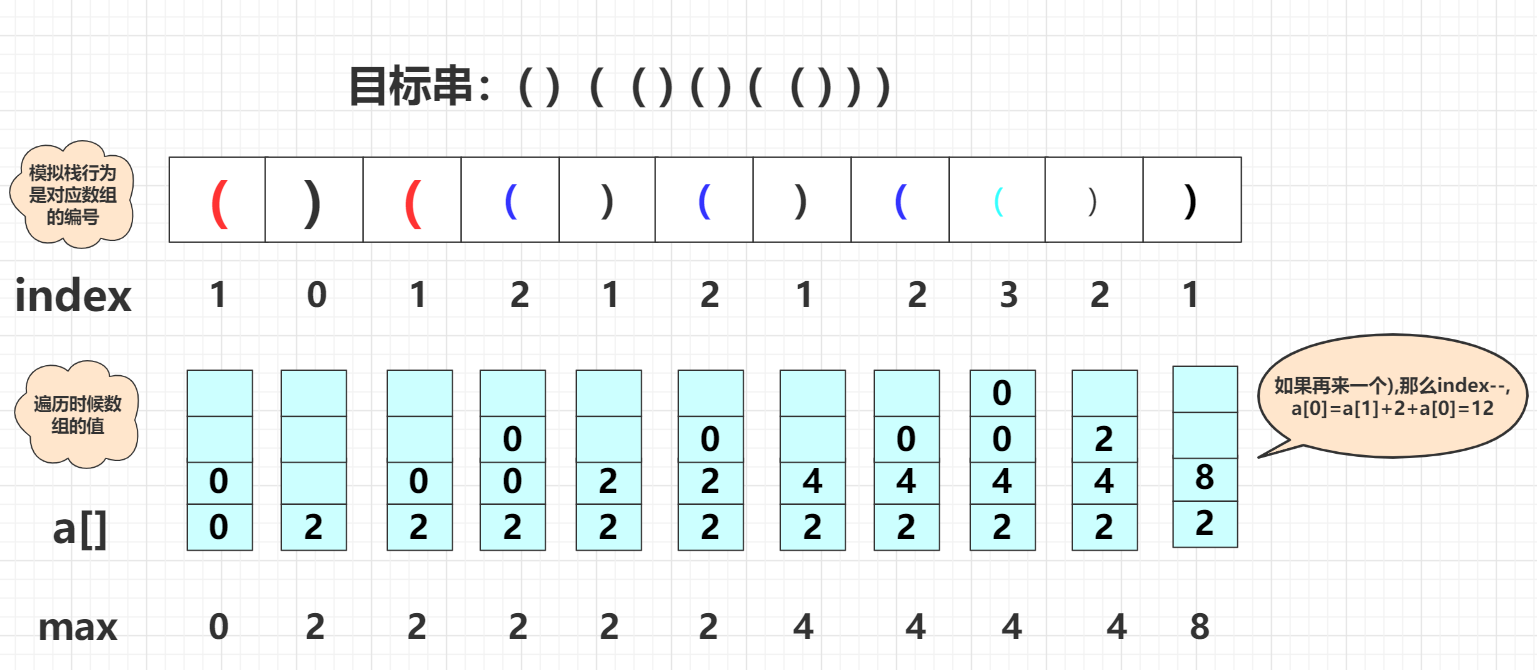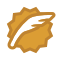# LeetCode 32最长有效括号(困难)

## 题目描述## 分析

public  int longestValidParentheses(String s) {
char str[]=s.toCharArray();//字符数组
int max=0;
for(int i=0;i<str.length-1;i++)
{
int index=-1;
if(max>=str.length-i)
break;
for(int j=i;j<str.length;j++)
{
if(str[j]=='(')
index++;
else {
if(index<0)
{
i=j;
break;
}
else {
index--;
}
}
if(index==-1&&(j-i+1>max))
{
max=j-i+1;
}
}
}
return max;
}• ( ) ) ( ) ( ( ) ( ) ) 最大为后面部分
• ( ) ( ) ( ( ( ) 最大为前面部分
• ( ( ( ( ( ( ) ( ) ( ) ( ) 最大为后面部分

(：左括号一旦出现那么他就期待一个)进行匹配，但它的后面可能有)并且在这中间有很多其他括号对。
):右扩号有两种情况：

• 一种是当前已经超过左括号前面已经不可能连续了。例如( ) ) ( )第三个括号出现已经使得整个串串不可能连续，最大要么在其左面要么再其右面。 你可以理解其为一种清零初始机制。
• 另一种情况)就是目标栈中存在(可与其进行匹配。匹配之后要叠加到消除后平级的数量上，并且判断是否是最大值。(下面会解释)

( ) ( ( ) ( ) ( ( ) ) )public static int longestValidParentheses(String s) {
int max=0;
int value[]=new int[s.length()+1];
int index=0;
for(int i=0;i<s.length();i++)
{
if(s.charAt(i)=='(')
{
index++;
value[index]=0;
}
else {//")"
if(index==0)
{
value=0;
}
else {
value[index-1]+=value[index--]+2;//叠加
if(value[index]>max)//更新
max=value[index];
}
}
}
return max;
}Big saiCSDN认证博客专家 数据结构与算法 爬虫 Java

07-119202
05-142604-23873
07-04382
04-02394
07-0557
02-1681
04-1853
04-14664
02-09192点击重新获取扫码支付余额充值